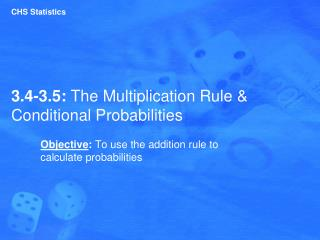Download Presentation3.4-3.5: The Multiplication Rule & Conditional Probabilities

# 3.4-3.5: The Multiplication Rule & Conditional Probabilities - PowerPoint PPT PresentationDownload Presentation## 3.4-3.5: The Multiplication Rule & Conditional Probabilities

- - - - - - - - - - - - - - - - - - - - - - - - - - - E N D - - - - - - - - - - - - - - - - - - - - - - - - - - -
##### Presentation Transcript

1. CHS Statistics 3.4-3.5: The Multiplication Rule & Conditional Probabilities Objective: To use the addition rule to calculate probabilities

2. Warm-up: Something to Consider… Consider the following two test questions: 1) True or False: Ms. Halliday’s favorite color is orange. 2) Ms. Halliday’s favorite sports team is: a) Pittsburgh Steelers b) Pittsburgh Penguins c) Baltimore Ravens d) New England Patriots e) Dallas Cowboys • If you do not know and you guess, what is the probability that you answer both questions correctly?

3. Independent vs. Dependent Events • Independent Events: when the outcome of one event does not affect the probability of the other event • Examples: • Rolling a 3 on a die, then rolling a 4 • Flipping a coin and getting heads, then flipping a coin again. • Dependent Events: when the outcome of one event affects the probability of the other event • Example: In the envelope activity, a student selected an envelope, and it was not replaced. The probabilities of the other events changed.

4. Examples: Dependent vs. Independent Decide whether the following events are independent or dependent: • Tossing a coin and getting a heads and then rolling a six-sided die and getting a 6 • Eating 10 cheeseburgers in a row and then getting a stomach ache

5. Experiment: Conditional Probability Experiment: Toss a coin. If you toss a heads you draw from Bag 1. Bag 1 contains 2 green marbles and 1 blue marble. If you toss a tails, you pick from Bag 2 which has 1 green marble and 3 blue marbles. • P(blue given tails was flipped)=

6. Conditional Probability • The probability of Event B occurring after it is assumed the Event A has already occurred. • P(B|A) is read as the probability of B given A.

7. Conditional Probability (cont.) Independence: • Independence of two events means that the outcome of one event does not influence the probability of the other. • With our new notation for conditional probabilities, we can now formalize this definition: • Events A and B are independent whenever P(B|A) = P(B). (Equivalently, events A and B are independent whenever P(A|B) = P(A).)

8. Multiplication Rule P(A and B) = P(A) ∙ P(B|A) • The probability of Event A times the Probability of Event B occurring, given Event A already occurred. • If your events are INDEPENDENT, your second probability won’t be affected by the first, so you would just multiply the two probabilities together. • If your events are DEPENDENT, you have to calculated the second, given that the first already occurred.

9. Examples: Multiplication Rule • A coin is tossed and a die is rolled. Find the probability of getting a heads and then rolling a 6. • Consider tossing a coin twice. What is the probability of getting 2 heads?

10. Examples: Multiplication Rule • The probability of a particular knee surgery is successful is 0.85. Find the probability that three knee surgeries will be successful. • Find the probability that none of three knee surgeries is successful.

11. Examples: Multiplication Rule • A bag contains 2 red cubes, 3 blue cubes, and 5 green cubes. If a red cube is removed, what is the probability that a green cube will be picked? • A pool of potential jurors consists of 10 men and 15 women. The Commissioner of Jurors randomly selects two names from this pool. Find the probability that the first is a man and the second is a man if two people are selected • With replacement • Without replacement

12. Conditional Probability Rule

13. Examples: Multiplication Rule • Find the probability of Event A occurs given that Event B already occurred. • P(2 spades) = • P(Even number on a die, given that the result of the die is 3 or less) = • P(Heart | Red) = • Find the probability of a couple having at least 1 girl among 3 children.

14. Examples: Contingency Tables The table below shows the results of a study where researchers examined a child’s IQ and the presence of a specific gene.   • Find the probability the child has a Normal IQ. • Find the probability that a child has a high IQ, given that the child has the gene.

15. Examples: Contingency Tables The table below shows the results of a study where researchers examined a child’s IQ and the presence of a specific gene.   • Find the probability that a child does not have the gene and has high IQ.  • Find the probability that a child does not have the gene, given that the child has a normal IQ. • Find the probability that a child has Normal IQ or Gene Present.

16. Let’s Look Back at the Warm-up: Consider the following two test questions: 1) True or False: Ms. Halliday’s favorite color is orange. 2) Ms. Halliday’s favorite sports team is: a) Pittsburgh Steelers b) Pittsburgh Penguins c) Baltimore Ravens d) New England Patriots e) Dallas Cowboys • If you do not know and you guess, what is the probability that you answer both questions correctly?

17. Assignment 1) pp. 140 – 144 # 1 – 3, 7, 9, 10, 16, 27 2) pp. 149 – 150 # 8, 17 – 20